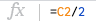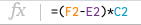# Equivalent Width Activity for EXES

## Searching for Europium using Stellar Spectroscopy

### The complete activity is meant to take a full class and maybe even two.The visible spectrum of the sun. Credit: OpenStax Astronomy. Fraknoi, Morrison, and Wolf

We are going to compare the relative amount of the elements nickel and europium in a couple of stars.

## Plotting A Stellar Spectrum

Watch the “HOW TO” video: https://www.youtube.com/watch?v=XkOUVOJrtrQ

2. Highlight the 2 columns of data. Most spreadsheets will assume the left column goes on the x-axis and the right column goes on the y-axis. This data is ready to go since column A is the wavelength in Angstroms and column B is the relative flux or amount of starlight. You can think of this as the brightness of a particular wavelength.
3. The easiest way to measure with the plot is if the chart type is set to ‘smooth line’.
4. Change the title of the graph to “Star name Spectrum” but use the real star name. 🙂
5. Change the y-axis title to Relative Flux or something similar.
6. Change the limits on the x-axis and y-axis so just the region with the absorption feature is visible.
7. Change the tick marks on the x-axis and y-axis so you can easily read off the plot. I used 0.05 on the y-axis with 4 minor marks and 0.1 on the x-axis with 4 minor marks. I also turned on all the various tick marks.

## How to measure equivalent width

1. First, count how far down from 1on the y-axis the “bell curve” bit goes and write that in a cell labeled Max Abs or something similar. This is the maximum absorption for this feature.2. Next, in an adjacent cell, hit = to enter a formula and click on the cell where you typed the value for the max absorption and divide that by 2. This is the half-max value.
3. We will use the half-max value to count down from 1 on the y-axis to where the curve meets that y-value on both the right and the left. Write down in an empty cell the left wavelength where the curve meets that half-max value and then write down in another empty cell the similar value on the right side of the curve.
4. Next, click an empty cell and click = to enter a formula. Be sure to put the difference between the right and left values in parentheses before you multiply by the max absorption.5. This value represents the equivalent width for this spectral feature. This is a numerical way to describe the relative abundance of a particular atom for a star. (Note: this is not the actual number of a particular atom.)
6. Repeat for the other stars!

## Plotting Spectra with Python and Google Colab

You can also try out some Python coding to plot the stellar spectra using Google Colab Notebook. Give it a try!